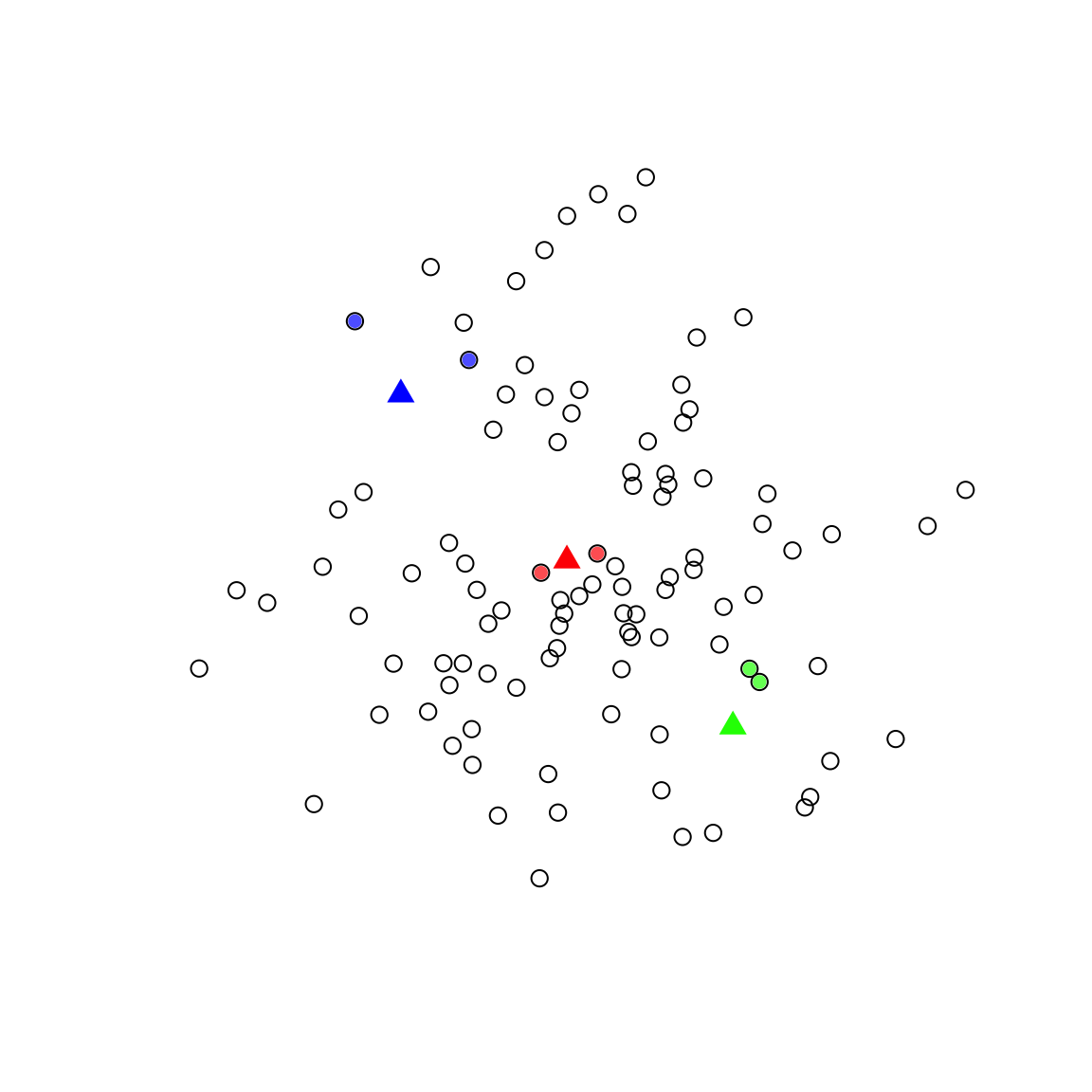First, my apologies – this is just a skeleton of a tutorial for now, but seems worth putting up even in this form!

# Nearest Neighbor

The naive way to find nearest neighbors is to use the gDistance function in the rgeos library to find the distance from each point in one dataset to each point in another. In small datasets, this works find, but it doesn’t scale – the number of calculations you need to do quickly explodes.

A better way is to use what’s called a “Spatial Index” – a very fancy algorithmic shortcut for limiting the number of calculations a computer needs to make.

R has a great library for this called SearchTrees, and you can easily use it to find a nearest neighbor (or in this case, the two nearest neighbors) as follows (with all credit to Josh O’Brien for this code snippet):

library(sp)
library(SearchTrees)

## Example data
set.seed(1)
A <- SpatialPoints(cbind(x = rnorm(100), y = rnorm(100)))
B <- SpatialPoints(cbind(x = c(-1, 0, 1), y = c(1, 0, -1)))

## Find indices of the two nearest points in A to each of the points in B.
## If you just want the one nearest neighbor, set k=1.
tree <- createTree(coordinates(A))
inds <- knnLookup(tree, newdat = coordinates(B), k = 2)

Wanna make sure it worked? Check it out!

## Show that it worked
plot(A, pch = 1, cex = 1.2)
points(B, col = c("blue", "red", "green"), pch = 17, cex = 1.5)
## Plot two nearest neigbors
points(A[inds[1, ], ], pch = 16, col = adjustcolor("blue", alpha = 0.7))
points(A[inds[2, ], ], pch = 16, col = adjustcolor("red", alpha = 0.7))
points(A[inds[3, ], ], pch = 16, col = adjustcolor("green", alpha = 0.7))